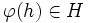# Proving that a subgroup is characteristic

Jump to: navigation, search
This survey article is about proof techniques for or related to satisfaction of the following property: characteristic subgroup
Find other survey articles about characteristic subgroup | Find fact articles that prove satisfaction of this property

This article explores the various ways in which, given a group and a subgroup (through some kind of description) we can try proving that the subgroup is a characteristic subgroup.

## Using the standard definitions

### Verifying invariance under all automorphisms

This definition states that$H$ is a characteristic subgroup of a group$G$ if and only if for every automorphism$\varphi$ of$G$ and every$h \in H$, we have that$\varphi(h) \in H$.

This definition could be used in three broad ways:

• Try everything: Here, we basically check every automorphism of$G$ and every element of$H$.
• Use generic elements: Here, we don't actually try each element, but rather, argue that for an arbitrary choice of automorphism of$G$ and element of$H$, the result holds.
• Use generating sets: This is a somewhat stronger version. It says that if$A$ is a generating set for$\operatorname{Aut}(G)$ and$B$ is a generating set for$H$, then it suffices to check that every element of$A \cup A^{-1}$ sends every element of$B$ to within$H$. In the case that$G$ is finite, we can check$A$ alone and don't need to check$A^{-1}$.

Note that we could hybridize the approaches, by, for instance, using generic elements on the automorphism side and generating sets on the subgroup side, or instead using generating sets on the automorphism side and generic elements on the subgroup side.

The generating set approach is also useful in computational situations, both when dealing with groups described by presentations and with groups described by means of generating sets inside larger ambient groups. For more on this, see characteristicity testing problem. It is not, however, very useful when proving abstract theorems about broad classes of groups. There, the generic elements approach is most likely to work well.

Here are some applications of the generic element approach:

Statement Statement with symbols Type Quick explanation of proof using this approach
Every group is characteristic in itself (proof section) For any group$G$,$G$ is characteristic in$G$ Subgroup metaproperty satisfaction for identity-true subgroup property Boils down to being definitionally true.
Trivial subgroup is characteristic In any group$G$, the trivial subgroup (the subgroup comprising only the identity element) is characteristic Subgroup metaproperty satisfaction for trivially true subgroup property Boils down to using the fact that the identity element is determined uniquely and cannot be moved by automorphisms.
Characteristicity is strongly intersection-closed If$H_i, i \in I$ are all characteristic in$G$, so is$\bigcap_{i \in I} H_i$ Subgroup metaproperty satisfaction for strongly intersection-closed subgroup property For$h \in \bigcap H$ and$\varphi \in \operatorname{Aut}(G)$, we have$h \in H_i$, so$\varphi(h) \in H_i$ for each$i$.

The generating set approach, or ideas of that kind, are more useful when the subgroup is described by means of generating elements or as a join of subgroups. The following are examples where we use the generating set approach on the subgroup side, but still uses the generic element approach on the automorphism side:

Statement Statement with symbols Type Quick explanation of proof using this approach
Characteristicity is strongly join-closed$H_i, i \in I$ characteristic in$G$, then so is the join of subgroups$\langle H_i \rangle_{i \in I}$ Subgroup metaproperty satisfaction Any element of the join is a product of elements in the$H_i$s. Use the invariance of each letter in the product. See also endo-invariance implies strongly join-closed
Characteristic subset generates characteristic subgroup If$A$ is a characteristic subset of$G$,$\langle A \rangle$ is a characteristic subgroup Random fact Write arbitrary element of$H$ as a product of elements of$A$ and their inverses, then apply the arbitrary automorphism.

## Subgroup-defining function

One of the simplest ways of showing that a subgroup is characteristic is to show that it arises from a subgroup-defining function. A subgroup-defining function is a rule that associates a unique subgroup to the group.

Any subgroup obtained via a subgroup-defining function is invariant under any automorphism of the group, and is hence a characteristic subgroup.

With this approach, for instance, we can show that the center, the derived subgroup, and the Frattini subgroup are characteristic.

## Starting from characteristic subgroups and using deterministic processes

An even more general idea than that of subgroup-defining functions is the following: any subgroup that is obtained by starting from a collection of characteristic subgroups and using deterministic processes, which may involve joins, intersections, centralizers, and commutators, or other processes, still yields a characteristic subgroup. Here, the term deterministic means invariant under automorphisms of the entire system.

## The deviation method of proving characteristicity

We could use either of these methods: